# Evaluating the boundary conditions for a rectangular pipe

guyvsdcsniper
Homework Statement:
Find the potential V (x,y) at all points across the section of this pipe.
Relevant Equations:
dv/dx=0
I have attached an image of the pipe in the attachmnts. The pipe is parallel to z-axis form (-∞,∞) and sides of length a.

So my boundary conditions for this problem are as follows
1.) V=0 at y=0
2.)V=0 at y=a
4.)∂v/∂x=0 @ x=0
3.)V0 @ x=a

I am a little confused on the fourth boundary condition only because my answer doesn't seem to match up with what I have found on the internet.

When solving B.C 4 and simplify i get, V0=Csin(nπy/a)(cosh(nπ)/2)

The answer I found gives V0=2Csin(nπy/a)(cosh(nπ))

Im not sure how they are getting the equation to be a multiple of 2 when the identity of (ex+e-x)=cosh(x)/2

The solution I found is the image that looks scanned. My work is the image that is in blue and black ink.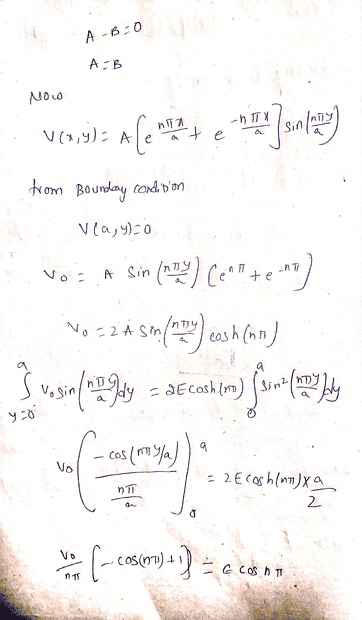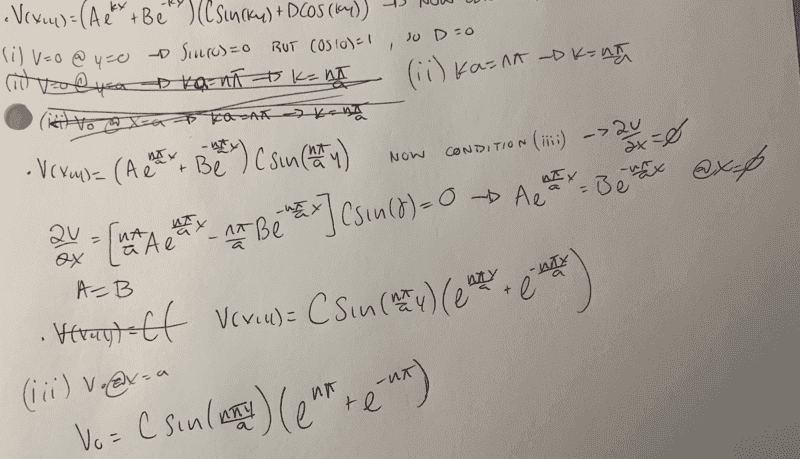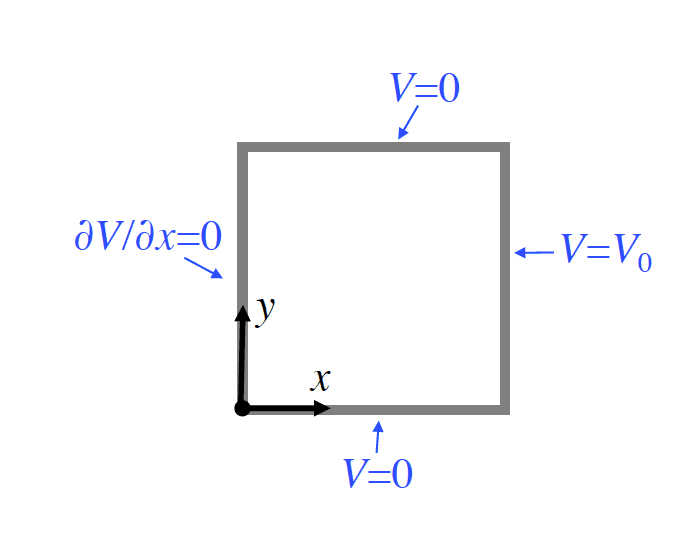Homework Helper
Gold Member
2022 Award
the identity of (ex+e-x)=cosh(x)/2
Are you quite sure about that?

•Orodruin
guyvsdcsniper
Are you quite sure about that?i totally messed that up. I swore I saw it like that when I looked that up last night.

guyvsdcsniper
So i finished solving the problem after correcting my mistake. I used the Fourier trick shown in my book to kind of help lead me through solving this problem.

I guess now is to just choose what n will be in the summation. I believe it should be odd non negative integers because if I have an even number for n, sin will be zero and the whole potential function will be zero.

Does this seem correct?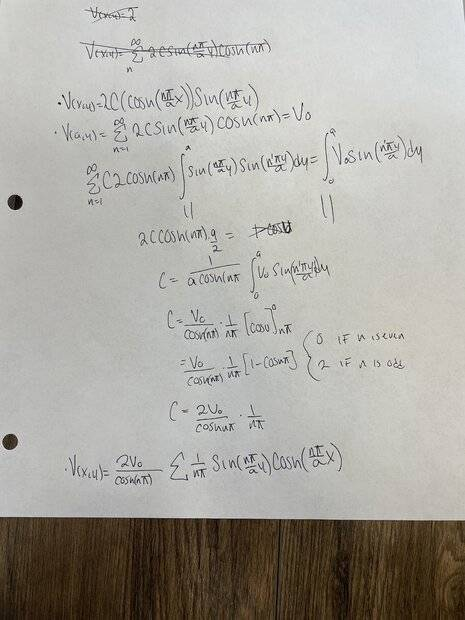Homework Helper
Gold Member
2022 Award
So i finished solving the problem after correcting my mistake. I used the Fourier trick shown in my book to kind of help lead me through solving this problem.

I guess now is to just choose what n will be in the summation. I believe it should be odd non negative integers because if I have an even number for n, sin will be zero and the whole potential function will be zero.

Does this seem correct?

View attachment 298142
Your answer doesn’t make sense because you have an n outside the summation.
You can always check the answer to such a question by seeing whether it satisfies all the equations you started with.
(I was also thrown at first by your notation, writing cosn for cosine instead of just cos.)

Staff Emeritus
Homework Helper
Gold Member
(I was also thrown at first by your notation, writing cosn for cosine instead of just cos.)
It is cosh, not cosn. Still, as you say, n cannot be outside the sum of course.

Homework Helper
Gold Member
2022 Award
It is cosh, not cosn. Still, as you say, n cannot be outside the sum of course.

Staff Emeritus
Homework Helper
Gold Member
To add to that, the sum is lacking any indication what the sum is taken over. Mid computation you have two cases (0 when n is even and 2 when n is odd), the sum should only be over odd n. Unless a sum is over all the range, it is very important to note what the sum is over.

Homework Helper
Gold Member
2022 Award
To add to that, the sum is lacking any indication what the sum is taken over. Mid computation you have two cases (0 when n is even and 2 when n is odd), the sum should only be over odd n. Unless a sum is over all the range, it is very important to note what the sum is over.
I think that part of @quittingthecult's question is how to determine the values to sum over. The reason for only using odd n offered in post #4 doesn’t look right to me.

guyvsdcsniper
To add to that, the sum is lacking any indication what the sum is taken over. Mid computation you have two cases (0 when n is even and 2 when n is odd), the sum should only be over odd n. Unless a sum is over all the range, it is very important to note what the sum is over.
Yea I can see having that cosh outside the summation is a big problem. Glaring mistake that I will fix.

I was holding out on identifying what n should be on the summation notation in hopes that I could get some feedback here before continuing.
My post may be a bit convoluted to follow as its not organized as good as can be,sorry about that.

So if I include that cosh into the summation (which I should of course, big mistake of mine). and you say the summation should only be when n is odd, but I have a sin(nπy/a). Letting n be odd is fine for cosh but would make sin go to zero which would make the potential go to zero.

So that's why I am confused about what interval n should be. Choosing odd or even essentially makes the potential go to zero

Homework Helper
Gold Member
2022 Award
Choosing odd or even essentially makes the potential go to zero
Everywhere? It's ##\sin(\frac{n\pi y}{a})##, not ##\sin({n\pi })##

guyvsdcsniper
Everywhere? It's ##\sin(\frac{n\pi y}{a})##, not ##\sin({n\pi })##
Dang i didnt think about the y. I guess does make a big difference.

So then my answer should be correct, as long as n is in the interval of even numbers in the summation?

guyvsdcsniper
I think this is the right answer, as long as I include the interval of n to be even non negative integers.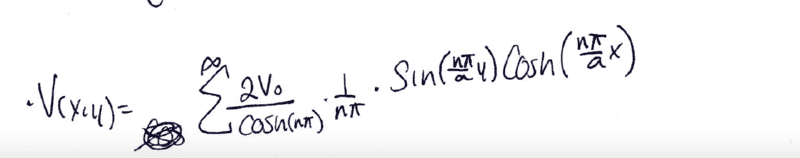My next task is to sketch the E field and Equipotential Contours in the pipe.

Honestly I am a little puzzled how to approach this. THis is my first time really dealing with this type of problem.

Homework Helper
Gold Member
2022 Award
include the interval of n to be even non negative integers.
Not quite sure what you mean by that. The question to settle is whether the set of values for n should be (nonnegative) even, odd or both.
Consider symmetry and the x=a boundary. Try n=1 and n=2.

guyvsdcsniper
Not quite sure what you mean by that. The question to settle is whether the set of values for n should be (nonnegative) even, odd or both.
Consider symmetry and the x=a boundary. Try n=1 and n=2.
Well when x=a, odd makes the potential negative and even makes the potential positive.

So I guess that means it can be even and odd ?

Homework Helper
Gold Member
2022 Award
Well when x=a, odd makes the potential negative and even makes the potential positive.

So I guess that means it can be even and odd ?
x=a is a boundary, with y ranging from 0 to a. What does that graph look like for n=1 and n=2?

Staff Emeritus
Homework Helper
Gold Member
I think that part of @quittingthecult's question is how to determine the values to sum over. The reason for only using odd n offered in post #4 doesn’t look right to me.
##1-\cos(\pi n) = 0## if ##n## is even seems pretty right to me.

Homework Helper
Gold Member
2022 Award
##1-\cos(\pi n) = 0## if ##n## is even seems pretty right to me.
Ah... I was looking at the text, which says "sin will be zero". It should say "C will be zero".

Staff Emeritus